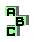CIRCUMCIRCLE    (4/13)

 r288 medial triangle, tangential triangle, inverse points r290 inverse points, altitude feet, point X(24), perspective r291 inverse points, point X(24), point X(403) r299 circumcenter, Kosnita point r301 circumcenter, medial triangle, Schoute point r311 incenter, isogonal points r317 concyclic points r318 similar triangles r323 incenter, intouch triangle, orthocenter, circumcenter r334 intouch triangle, orthic triangle, homothetic triangles r359 incenter, tangent circles r375 ninecircle, point X(115), incentric triangle, Feuerbach point r385 orthocenter, Steiner circumellipse, inconic, perspector r406 centroid, incenter, excentral triangle, point X(165) r407 Euler line, cevian triangle, circumconic, isogonal points, point X(115), point X(125), inverse points r408 cevian triangle, isogonal points, complement, inverse points r426 orthocenter, circumcenter, cevian triangle d point X(468) d far-out point d Schröder point d Bevan-Schröder point r445 excircles, perspective, Clawson point r446 circumconic, point X(100), point X(190), Mittenpunkt1 2 3 4 5 6 7 8 9 10 11 12 13